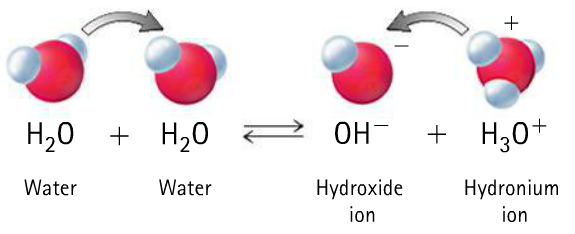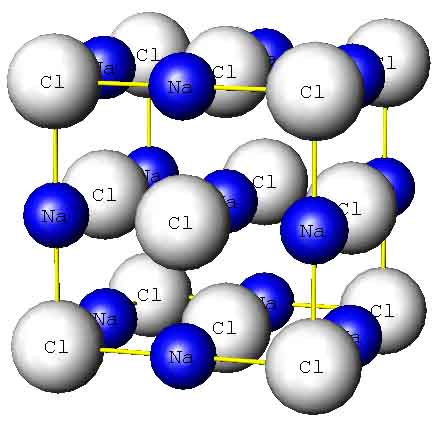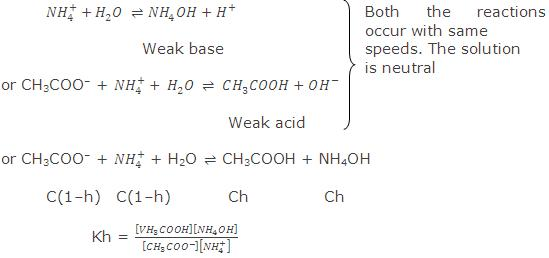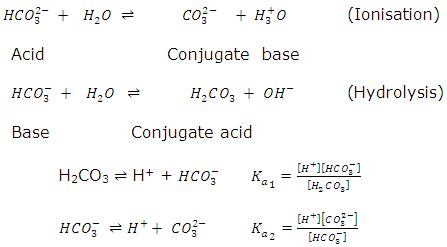×#### Thank you for registering.

One of our academic counsellors will contact you within 1 working day.

Click to Chat

1800-1023-196

+91-120-4616500

CART 0

• 0

MY CART (5)

Use Coupon: CART20 and get 20% off on all online Study Material

ITEM
DETAILS
MRP
DISCOUNT
FINAL PRICE
Total Price: Rs.

There are no items in this cart.
Continue ShoppingSalt Hydrolysis

Table of Content

Salt of a Strong Acid and a Weak Base

Relation Between Hydrolysis Constant and Degree of Hydrolysis

Salt of a Weak Acid and a Strong Base

Salt of a Weak Acid and a Weak Base

Hydrolysis of Amphiprotic Anion

Hydrolysis at a Glance

Related ResourcesPure water is a weak electrolyte. Weak because it does not completely disassociates  into hydrogen and hydroxide but exist in equilibrium with these two ions. It is  neutral in nature, i.e., H+ ion concentration is exactly equal to OH- ion concentration

[H+] = [OH-]When this condition is disturbed by decreasing the concentration of either of the two ions, the neutral nature changes into acidic or basic. When [H+] > [OH-], the water becomes acidic and when [H+] < [OH-], the water acquires basic nature. This is exactly the change which occurs during the phenomenon known as salt hydrolysis. It is defined as a reaction in which the cation or anion or both of a salt react with water to produce acidity or alkalinity.Salts are strong electrolytes. When dissolved in water, they dissociate almost completely into ions. In some salts, cations are more reactive in comparison to anions and these react with water to produce H+ ions. Thus, the solution acquires acidic nature.

M+ +   H2O    MOH    +  H+

Weak base

In other salts, anions may be more reactive in comparison to cations and these react with water to produce OH- ions. Thus, the solution becomes basic.

A- +   H2O     HA   +  OH-

Weak acid

The process of salt hydrolysis is actually the reverse of neutralization.

Salt + Water   Acid + Base

If acid is stronger than base, the solution is acidic and in case base is stronger than acid, the solution is alkaline. When both the acid and the base are either strong or weak, the solution is generally neutral in nature.

As the nature of the cation or the anion of the salt determines whether its solution will be acidic or basic, it is proper to divide the salts into four categories.

Salt of a strong acid and a weak base.
Examples:  FeCl3, CuCl2, AlCl3, NH4Cl, CuSO4, etc.

Salt of a strong base and a weak acid.
Examples: CH3COONa, NaCN, NaHCO3, Na2CO3, etc.

Salt of a weak acid and a weak base.
Examples: CH3COONH4, (NH4)2CO3, NH4HCO3, etc.

Salt of a strong acid and a strong base.
Examples: NaCl, K2SO4, NaNO3, NaBr, etc.Refer to the following video for salt hydrolysis

Salt of a Strong Acid and a Weak Base

The solution of such a salt is acidic in nature. The cation of the salt which has come from weak base is reactive. It reacts with water to form a weak base and H+ ions.

B+  +   H2O    BOH  +  H+

Weak base

Consider, for example, NH4Cl. It ionises in water completely into NH4 and CF ions. ions react with water to form a weak base (NH4OH) and H+ ions.

NH+4  +  H2O      NH4OH  +  H+

C(1-x)                   Cx         Cx

Thus, hydrogen ion concentration increases and the solution becomes acidic.

Applying law of mass action,

...... (i)

where C is the concentration of salt and x the degree of hydrolysis.

Other equilibria which exist in solution are

NH4OH  NH+4 + OH-,

.... (ii)

H2O   H+ + OH-,

Kb = [H+][H-]            ..... (iii)

From eqs. (II) and (iii)

Kw/Kb =[H+ ][NH4 OH]/[NH4+ ] = Kh      .... (iv)

[H+] = [H+ ][NH4+]/[NH4OH] = Kw/Kb ×[NH4+ ]/[NH4 OH]

log [H+] = log Kw - log Kb + log[salt]/[base]

-pH = -pKw + pKb + log[salt]/[base]

pKw - pH = pKb + log[salt]/[base]

Relation Between Hydrolysis Constant and Degree of Hydrolysis

The extent to which hydrolysis proceeds is expressed as the degree of hydrolysisand is defined as the fraction of one mole of the salt that is hydrolysed when the equilibrium has been attained. It is generally expressed as h or x.

h = (Amount of salt hydrolysed)/(Total salt taken)

Considering again eq. (i),

Kh = x2C/(1-x)   or     Kh = h2C/(1-h)

When h is very small (1-h) → 1,

H2 = Kh × 1/c

or   h = √(Kh/C)  = √(Kw/Kb  C)

[H+] = h × C = √(C  Kh)/Kb

log [H+] =  1/2 log Kw + 1  1/2log C - 1/2log Kb

Salt of a Weak Acid and a Strong BaseThe solution of such a salt is basic in nature. The anion of the salt is reactive. It reacts with water to form a weak acid and OH- ions.

A- + H2O;      HA + OH-

Weak acid

Consider, for example, the salt CH3COONa. It ionises in water completely to give CH3COO- and Na+ ions. CH3COO- ions react with water to form a weak acid, CH3COOH and OH- ions.

CH3COO- + H2O     CH3COOH + OH-

C(1-x)                         Cx           Cx

Thus, OH- ion concentration increases, the solution be­comes alkaline.

Applying law of mass action,

Kh = [CH3COOH][OH-]/[CH3CO-] = (Cx×Cx)/C(1-x) = (Cx2)/(1-x) )  ...... (i)

Other equations present in the solution are:

CH3COOH  CH3COO- + H+,

Ka = [CH3COO-][H+]/[CH3COOH]      ...... (ii)

H2O   H+ + OH-,

Kw = [H+][OH-]    ....... (iii)

From eqs. (ii) and (iii),

log [OH-] = log Kw - log Ka + log[salt]/[acid]

-pOH = -pKw + pKa + log[salt]/[acid]

pKw - pOH = pKa + log[salt]/[acid]

Considering eq. (i) again,

Kh = cx2/(1-x)      or    Kh = Ch2/(1-h)

When h is very small, (1-h) → 1

or     h2 = Kh/C

or     h =  √Kh/C

[OH-] = h × C = √(CKh) = √(C  Kw/Ka)

[H+] = Kw/[OH-]

= Kw/√(C*Kw/Ka) = √(Ka Kw)/Kc

-log [H+] = -1/2log Kw - 1/2log Ka + 1/2log C

pH =  1/2pKw +  1/2pKa +  1/2log C

= 7 + 1/2pKa +  1/2log C.

Salt of a Weak Acid and a Weak BaseMaximum hydrolysis occurs in the case of such a salt as both the cation and anion are reactive and react with water to produce H+ and OFT ions. The solution is generally neutral but it can be either slightly acidic or slightly alkaline if both the reactions take place with slightly different rates. Consider, for example, the salt CH3COONH4. It gives CH3COO- and  ions in solution. Both react with water.Other equilibria which exist in solution are:

CH3COOH   CH3COO- + H+,

Ka =  [CH3COO-][H+]/[CH3COOH]    ..... (i)

NH4OH  NH+4 + OH-,

Kb = [NH+4] [OH-]/[NH4OH]           ..... (ii)

H2O  H+ + OH-

Kw = [H+][OH-]                                    ..... (iii)

From Eqs. (i), (ii) and (iii),

Kh =  Kw/Ka.Kb = [CH3COOH][NH4OH]/[CH3COO-][NH+4]                     .... (iv)

Let C be the concentration and h be the degree of hydrolysis

Kh = h2/(1-h)2

When h is small, (1-h) → 1.

Kh = h2

h = √Kh = √Kw/Ka*Kb

[H+] Ka × h

= Ka × √Kw/Ka*Kb

= √Kw  Ka/Kb

-log [H+] = -1/2log Ka - 1/2log Kw + 1/2log Kb

pH = 1/2pKa +  1/2pKw - 1/2pKb

= 7 +  1/2pKa - 1/2pKb

When pKa = pKb, pH = 7, i.e., solution will be neutral in nature.

When pKa > pKb. The solution will be alkaline as the acid will be slightly weaker than base and pH value will be more than 7.

In case pKa < pKb, the solution will be acidic as the acid is relatively stronger than base and pH will be less than 7.

Salt of a Strong Acid and a Strong Base

Such a salt, say NaCl, does not undergo hydrolysis as both the ions are not reactive. The solution is thus, neutral in nature.

Hydrolysis of Amphiprotic Anion

Let us consider hydrolysis of amphiprotic anion only, i.e., when counter cation is not hydrolysed, example of some salts of this category are NaHCO3, NaHS, Na2HPO4, NaH2PO4.Here, H2PO-4 and HPO2-4  are amphiprotic anions. pH after their hydrolysis can be calculated as,

pH of  H2PO-4  in aqueous medium = (pka1 + pka2)/2

pH of H2PO2-4  in aqueous medium = (pka2 + pka3)/2

Here, H2PO2-4  is conjugate base of H2PO-4 and H3PO4 is conjugate acid of H2PO-4.

Similarly, PO3-4 is conjugate base of HPO-24  and HPO-4  is conjugate acid of PO3-4 .

(iv)  Let us consider amphiprotic bicarbonate anion.pH HCO-3of  ion after hydrolysis in aqueous medium  = (pka1 + pka2)/2

(v)  Let us consider the hydrolysis of amphiprotic anion along with cation, e.g., NH4HCO3, NH4HS.

In above examples both cations and anions are derived from weak base and weak acids respectively hence, both will undergo hydrolysis in aqueous medium.

When these salts are dissolved in water, [H3O+] concentra­tion can be determined as,

[H3O+] = √ka1[kw/kb + ka2]

pH = -log = √ka1[kw/kb + ka2]

Hydrolysis at a Glance

Salt

Nature

Degree

Hydrolysis Constant

pH

NaCl

(Strong acid + Strong Base)

2. Ch3COONa (Weak acid + Strong base)

3. NH4Cl

(Strong acid + Weak base)

4. CH3COONH4   (Weak     acid + Weak base)

Neutral

Base

Acidic

*

No Hydrolysis

h = √kw/Cka

h = √kw/Ckb

h = √kw/(ka + kb)

-

Kh = kw/ka

Kh = kw/Ckb

Kh = kw/(ka + kb)

-

pH=1/2[pkw + pka + logC]

pH=1/2[pkw- pkb - logC]

pH=1/2[pkw + pka - pkb]

In the case of salt of weak acid and weak base, nature of medium after hydrolysis is decided in the following manner:

(i)    If Ka = Kb, the medium will be neutral.

(ii)  If Ka > Kb, the medium will be acidic.

(iii)  If Ka < Kb, the medium will be basic.

The degree of hydrolysis of salts of weak acids and weak bases is unaffected by dilution because there is no concentration term in the expression of degree ofhydrolysis.

Note : Degree of hydrolysis always increases with increase in temperature because at elevated temperature increase in Kw is greater as compared to Ka and Kb.

Related Resources

JEE  Syllabus

Reference Books for IIT JEE

Solubility Product

To read more, Buy study materials of Chemical Equilibrium comprising study notes, revision notes, video lectures, previous year solved questions etc. Also browse for more study materials on Chemistry here.### Course Features

• 731 Video Lectures
• Revision Notes
• Previous Year Papers
• Mind Map
• Study Planner
• NCERT Solutions
• Discussion Forum
• Test paper with Video Solution